# The Runge Phenomenon and Chebyshev interpolation¶

## A polynomial approximation to $f(x) = \sin(x)$¶

Here we'll use four easily computable data points to construct a polynomial approximation to $f(x) = \sin(x)$. We know

\begin{array}{c|c} x_i & y_i = \sin(x_i) \\ 0 & 0 \\ \pi/6 & 1/2 \\ \pi/3 & \sqrt{3}/2 \\ \pi/2 & 1 \end{array}
In :
include("polyinterp.jl")
using PyPlot

In [ ]:


In :
xi = [0 π/6 π/3 π/2]
yi = [0 1/2 sqrt(3)/2 1]
(c,b) = newtondivdiff(xi,yi)
x = linspace(-π/6, 4π/6)
p = polyeval(c,b,x)
clf()
plot(xi,yi,"bo")
plot(x, p, "r-")
plot(x,sin(x),"b-")
xlabel("x")
legend(("(xi,yi)", "P(x)", "sin x"), loc="upper left")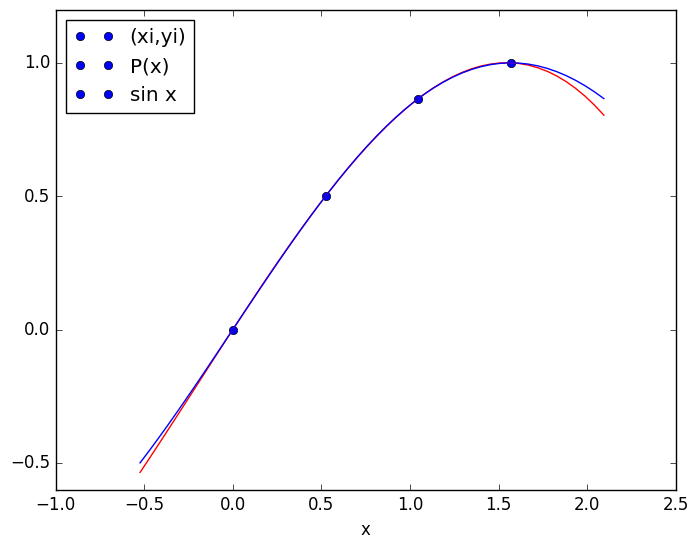Out:
PyObject <matplotlib.legend.Legend object at 0x7f50be736f50>
WARNING: Base.writemime is deprecated.
likely near /home/gibson/.julia/v0.5/IJulia/src/kernel.jl:31
WARNING: Base.writemime is deprecated.
likely near /home/gibson/.julia/v0.5/IJulia/src/kernel.jl:31
WARNING: Base.writemime is deprecated.
likely near /home/gibson/.julia/v0.5/IJulia/src/kernel.jl:31
WARNING: Base.writemime is deprecated.
likely near /home/gibson/.julia/v0.5/IJulia/src/kernel.jl:31
WARNING: Base.writemime is deprecated.
likely near /home/gibson/.julia/v0.5/IJulia/src/kernel.jl:31
WARNING: Base.writemime is deprecated.
likely near /home/gibson/.julia/v0.5/IJulia/src/kernel.jl:31
WARNING: Base.writemime is deprecated.
likely near /home/gibson/.julia/v0.5/IJulia/src/kernel.jl:31
WARNING: Base.writemime is deprecated.
likely near /home/gibson/.julia/v0.5/IJulia/src/kernel.jl:31
WARNING: Base.writemime is deprecated.
likely near /home/gibson/.julia/v0.5/IJulia/src/kernel.jl:31
WARNING: Base.writemime is deprecated.
likely near /home/gibson/.julia/v0.5/IJulia/src/kernel.jl:31
WARNING: Base.writemime is deprecated.
likely near /home/gibson/.julia/v0.5/IJulia/src/kernel.jl:31
WARNING: Base.writemime is deprecated.
likely near /home/gibson/.julia/v0.5/IJulia/src/kernel.jl:31
WARNING: Base.writemime is deprecated.
likely near /home/gibson/.julia/v0.5/IJulia/src/kernel.jl:31
in show at /home/gibson/.julia/v0.5/PyCall/src/PyCall.jl
WARNING: Base.writemime is deprecated.
likely near /home/gibson/.julia/v0.5/IJulia/src/kernel.jl:31
in show at /home/gibson/.julia/v0.5/PyCall/src/PyCall.jl
WARNING: Base.writemime is deprecated.
likely near /home/gibson/.julia/v0.5/IJulia/src/kernel.jl:31
in show at /home/gibson/.julia/v0.5/PyCall/src/PyCall.jl
WARNING: Base.writemime is deprecated.
likely near /home/gibson/.julia/v0.5/IJulia/src/kernel.jl:31
in show at /home/gibson/.julia/v0.5/PyCall/src/PyCall.jl
WARNING: Base.writemime is deprecated.
likely near /home/gibson/.julia/v0.5/IJulia/src/kernel.jl:31
in show at /home/gibson/.julia/v0.5/PyCall/src/PyCall.jl
WARNING: Base.writemime is deprecated.
likely near /home/gibson/.julia/v0.5/IJulia/src/kernel.jl:31
in show at /home/gibson/.julia/v0.5/PyCall/src/PyCall.jl
WARNING: Base.writemime is deprecated.
likely near /home/gibson/.julia/v0.5/IJulia/src/kernel.jl:31
in show at /home/gibson/.julia/v0.5/PyCall/src/PyCall.jl
WARNING: Base.writemime is deprecated.
likely near /home/gibson/.julia/v0.5/IJulia/src/kernel.jl:31
in show at /home/gibson/.julia/v0.5/PyCall/src/PyCall.jl
WARNING: Base.writemime is deprecated.
likely near /home/gibson/.julia/v0.5/IJulia/src/kernel.jl:31
in show at /home/gibson/.julia/v0.5/PyCall/src/PyCall.jl
WARNING: Base.writemime is deprecated.
likely near /home/gibson/.julia/v0.5/IJulia/src/kernel.jl:31
in show at /home/gibson/.julia/v0.5/PyCall/src/PyCall.jl
WARNING: Base.writemime is deprecated.
likely near /home/gibson/.julia/v0.5/IJulia/src/kernel.jl:31
in show at /home/gibson/.julia/v0.5/PyCall/src/PyCall.jl
WARNING: Base.writemime is deprecated.
likely near /home/gibson/.julia/v0.5/IJulia/src/kernel.jl:31
in show at /home/gibson/.julia/v0.5/PyCall/src/PyCall.jl

In :
plot(xi, [0 0 0 0], "bo")
plot(x, sin(x)-p, "r-")
xlabel("x")
legend(("datapts", "sin x - P(x)"))
grid("on")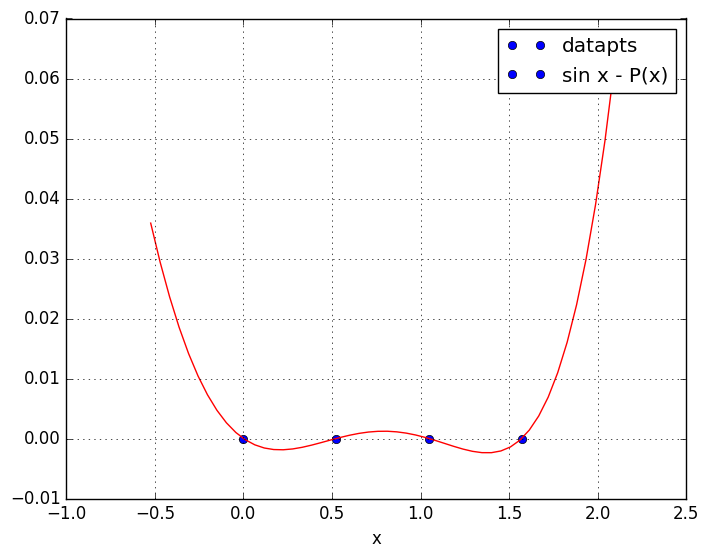In :
sin(1) - polyeval(c,b,1) # evaluate sin(x) - P(x) at x=1

Out:
0.0003849684673111753

## Polynomial interpolant of triangular bump, uniform gridpoints¶

In :
xi = linspace(-1.0, 1.0, 13)
yi = [0, 0, 0, 0, 0, 0, 1.0, 0, 0, 0, 0, 0, 0]
plot(xi,yi,"bo")
ylim(-1.1, 1.1)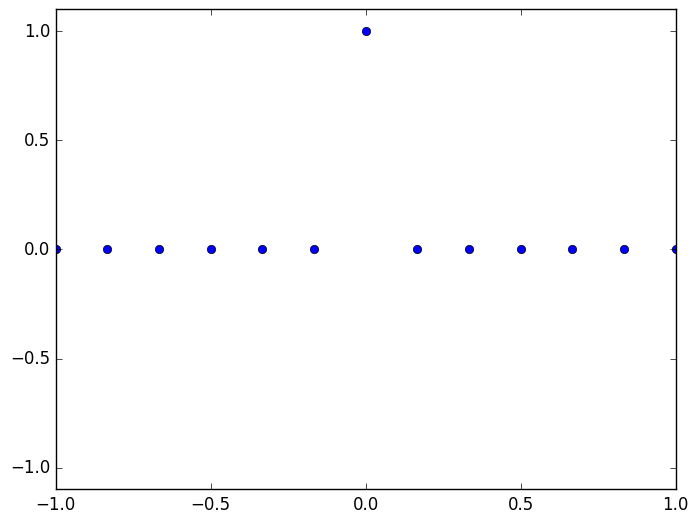Out:
(-1.1,1.1)
In :
xi = linspace(-1.0, 1.0, 13)
yi = [0, 0, 0, 0, 0, 0, 1.0, 0, 0, 0, 0, 0, 0]
plot(xi,yi,"bo")

(c,b) = newtondivdiff(xi,yi)
x = linspace(-1.2,1.2,200)
p = polyeval(c,b,x)
plot(x,p, "r-")

xlabel("x")
legend(("datapts", "P(x)"))
ylim((-5,5))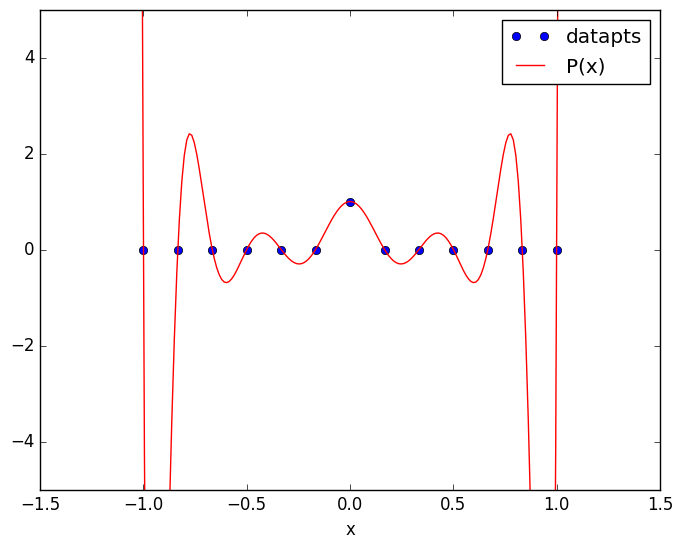Out:
(-5,5)

## Polynomial interpolation of Runge Example Function, uniform gridpoints¶

The Runge Example Function $f(x) = 1/(1+12x^2)$ is like a smoother form of the triangular bump, but it's still problematic for polynomial interpolation.

In :
f(x) = 1./(1+12x.^2)
x = linspace(-1,1,200)
plot(x,f(x),"b-")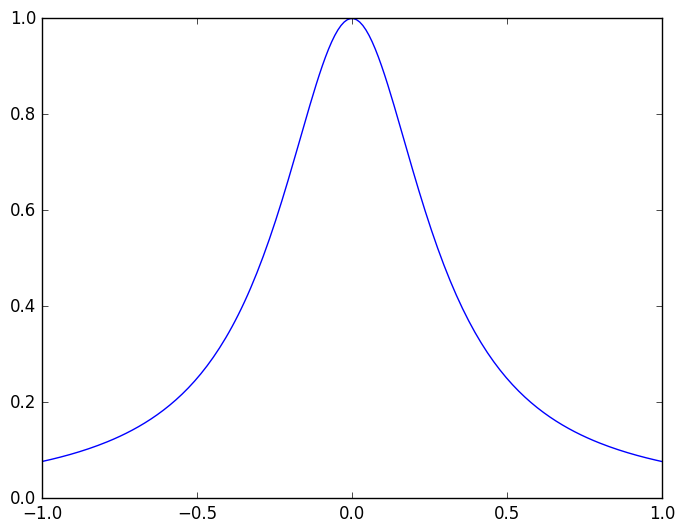Out:
1-element Array{Any,1}:
PyObject <matplotlib.lines.Line2D object at 0x7f50bdf46fd0>

Interpolate the Runge Example Function with 15 data points on [-1, 1]

In :
xi = linspace(-1,1,15)
yi = f(xi)
(c,b) = newtondivdiff(xi,yi)
x = linspace(-1,1,200)
p = polyeval(c,b,x)
plot(xi,yi, "bo")
plot(x,p, "r-")
xlabel("x")
xlim((-1.1, 1.1))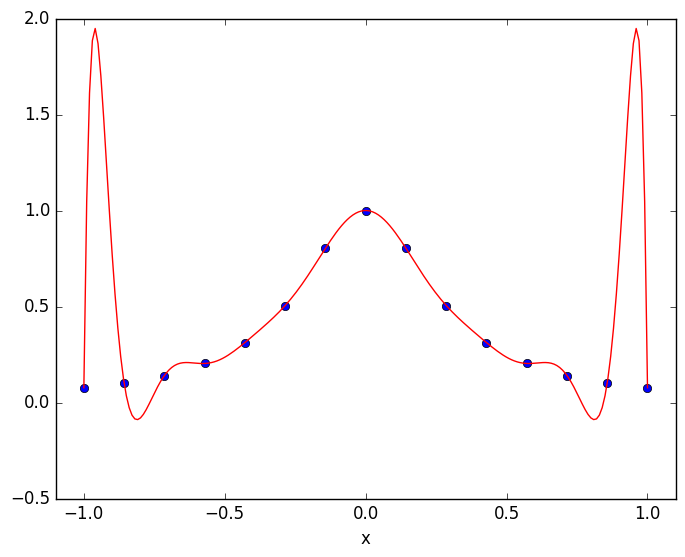Out:
(-1.1,1.1)
In :
plot(xi,0*xi, "bo")
plot(x,f(x)-p, "r-")
xlabel("x")
xlim((-1.1, 1.1))
title("interpolation error f(x) - p(x)")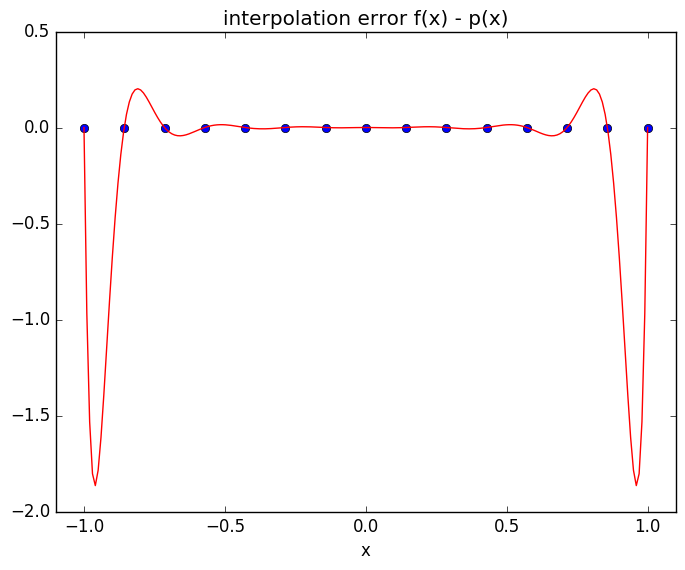Out:
PyObject <matplotlib.text.Text object at 0x7f50bde7f290>

Same with twice as many datapoints

In :
xi = linspace(-1,1,31)
yi = f(xi)
(c,b) = newtondivdiff(xi,yi)
x = linspace(-1,1,200)
p = polyeval(c,b,x)
plot(xi,yi, "bo")
plot(x,p, "r-")
xlabel("x")
xlim((-1.1, 1.1))
ylim((-2,2))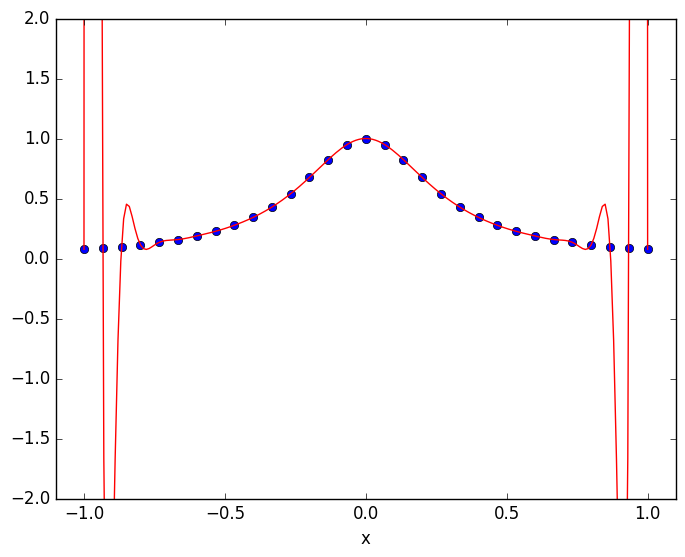Out:
(-2,2)
In [ ]:
plot(xi,yi, "bo")
plot(x,p, "r-")
xlabel("x")
xlim((-1.1, 1.1))
ylim((-2,2))


The Runge Phenomenon: Increasing the density of uniform data points does not improve the interpolant near the endpoints. In fact it makes it worse!

## The error polynomial¶

In :
# define error polynomial for base points xi
function E(x,xi)
p = ones(length(x))
n = length(xi)
for i = 1:n
p = p.*(x-xi[i])
end
p /= factorial(float(n))
end

Out:
E (generic function with 1 method)
In :
xi = linspace(-1,1,17)
x = linspace(-1,1,200)
plot(xi,xi*0,"bo")
plot(x,E(x,xi), "r-")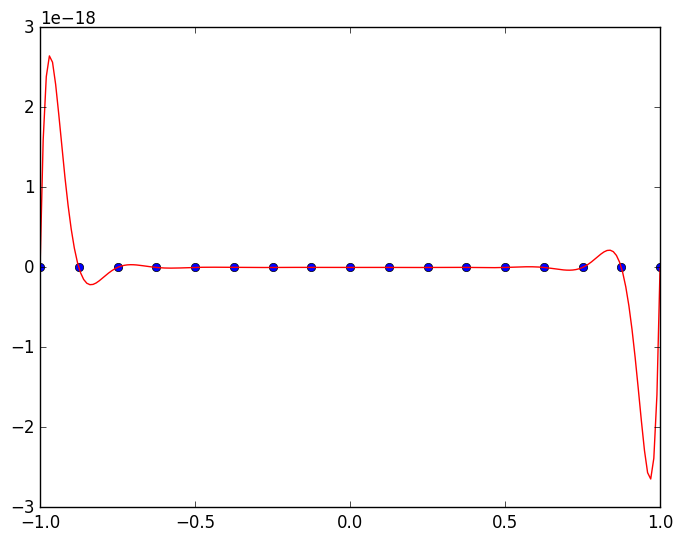Out:
1-element Array{Any,1}:
PyObject <matplotlib.lines.Line2D object at 0x7f50be013790>
In [ ]:
xi


The Runge phenomenon is caused by the error polynomial being larger near the endpoints.

## Chebyshev points¶

To improve the behavior of the interpolant near the endpoints, use denser datapoints near ends than in middle. Optimum distribution is given by the Chebyshev points

\begin{equation*} x_i = \cos \frac{(2i-1)\pi}{2n} \quad \text{for } i = 1, \ldots, n \end{equation*}
In :
n=17
xi = cos((2*(1:n)-1)π/(2n))
plot(xi,0*xi, "bo")
plot(x, E(x,xi), "r-")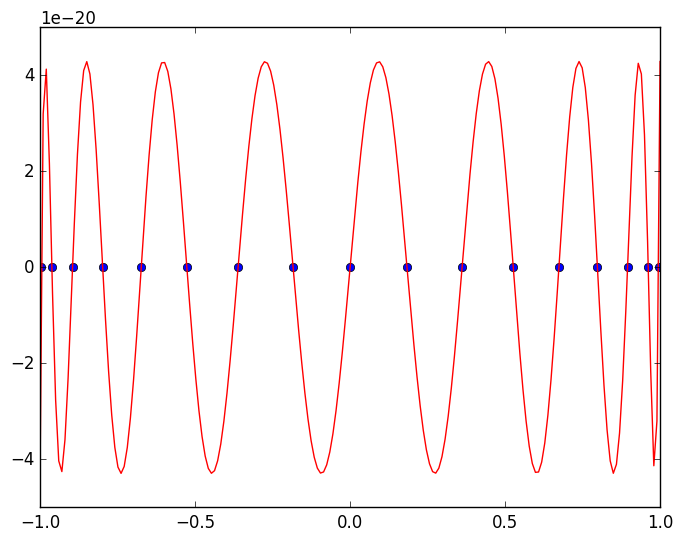Out:
1-element Array{Any,1}:
PyObject <matplotlib.lines.Line2D object at 0x7f50be4f9250>
In :
n=17
xi = cos((2*(1:n)-1)π/(2n))
yi = f(xi)
plot(xi,yi,"bo")
(c,b) = newtondivdiff(xi,yi)
x = linspace(-1.1, 1.1, 200)
p = polyeval(c,b,x)
plot(xi,yi,"bo")
plot(x,p,"r-")
plot(x,f(x),"b-")
ylim((-2,2))
grid("on")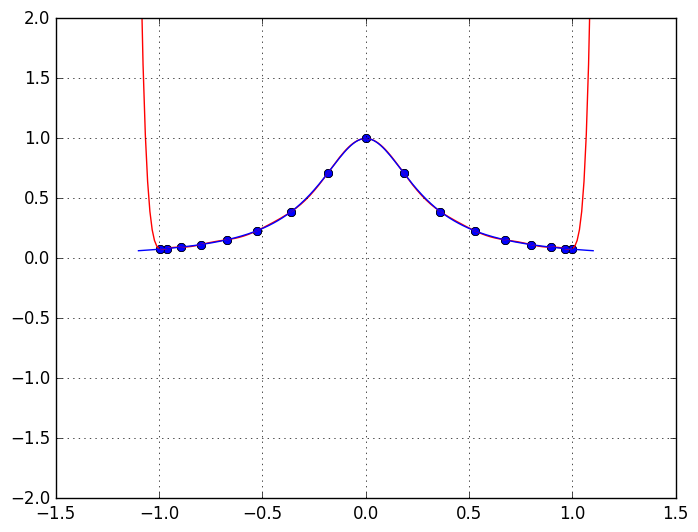In :
n=31
xi = cos((2*(1:n)-1)π/(2n))
yi = f(xi)
plot(xi,yi,"bo")
(c,b) = newtondivdiff(xi,yi)
x = linspace(-1.1, 1.1, 200)
p = polyeval(c,b,x)
plot(xi,yi,"bo")
plot(x,p,"r-")
plot(x,f(x),"b-")
ylim((-2,2))
grid("on")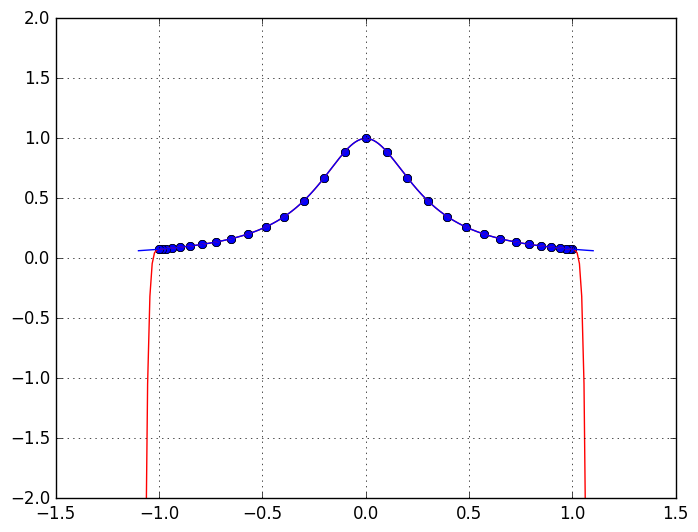In :
plot(x,f(x)-p,"r-")
ylim(-0.001,0.001)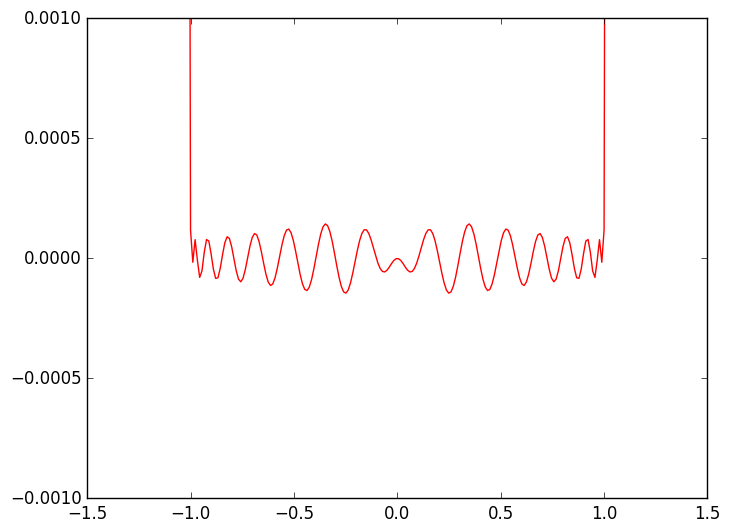Out:
(-0.001,0.001)
In [ ]: